# Catch up on the latest AI articles# Can Transformer Revolutionize Reinforcement Learning This Time?

3 main points
✔️ Decision Transformer that treats reinforcement learning as a simultaneous probability distribution series modeling with an autoregressive model
✔️ An architecture that is a simple adaptation of GPT's Causal Transformer
✔️ Achieve SOTA for Model-free methods in the Offline RL setting

written by Lili ChenKevin LuAravind RajeswaranKimin LeeAditya GroverMichael LaskinPieter AbbeelAravind SrinivasIgor Mordatch
(Submitted on 2 Jun 2021 (v1), last revised 24 Jun 2021 (this version, v2))

Subjects:  Machine Learning (cs.LG); Artificial Intelligence (cs.AI)code：## Introduction: Can Transformer revolutionize RL as well?

Recent work, including the Zero-shot language generation model GPT-3 and the out-of-distribution image generation model DALL-E, has shown that Transformers can learn higher-order semantic concepts, inspired by the fact that NLP-born Transformers are very active in the CV world. Can Transformer revolutionize the world of reinforcement learning as well? In this article, we introduce research that has the potential to do just that.

There have been previous studies that attempted to apply promising transformers to reinforcement learning. Unlike them, this work proposes a Decision Transformer that treats the simultaneous probability distributions of state, action, and reward as autoregressive models, changing the way reinforcement learning is handled. In the Offline RL problem setting, it achieves accuracy comparable to existing model-free SOTA methods.

In this section, we will explain the Decision Transformer using toy data to understand it intuitively.

Consider the task of finding the shortest path in the effective graph shown in Figure 2 (left). As a reinforcement learning problem, we set the reward to 0 if the agent reaches the goal node, and -1 otherwise. Using the idea of return-to-go, a dataset like Figure.2 (middle) can be obtained by random measures. For example, a node with -2 means that the goal node is reached after two steps in the trajectory data. Here I trained an autoregressive model that predicts the next token using the language generation model GPT for a series of data with state, action, and future earnings as a single token. At the time of evaluation, we let the system predict the next node (action) to go based on the current time state, the target reward, and past information. As a result, we were able to predict the action that corresponds to the token whose state and target reward pair are related from the training data set. Figure 2 (right) also shows that we can learn better strategies than simply mimicking the training data.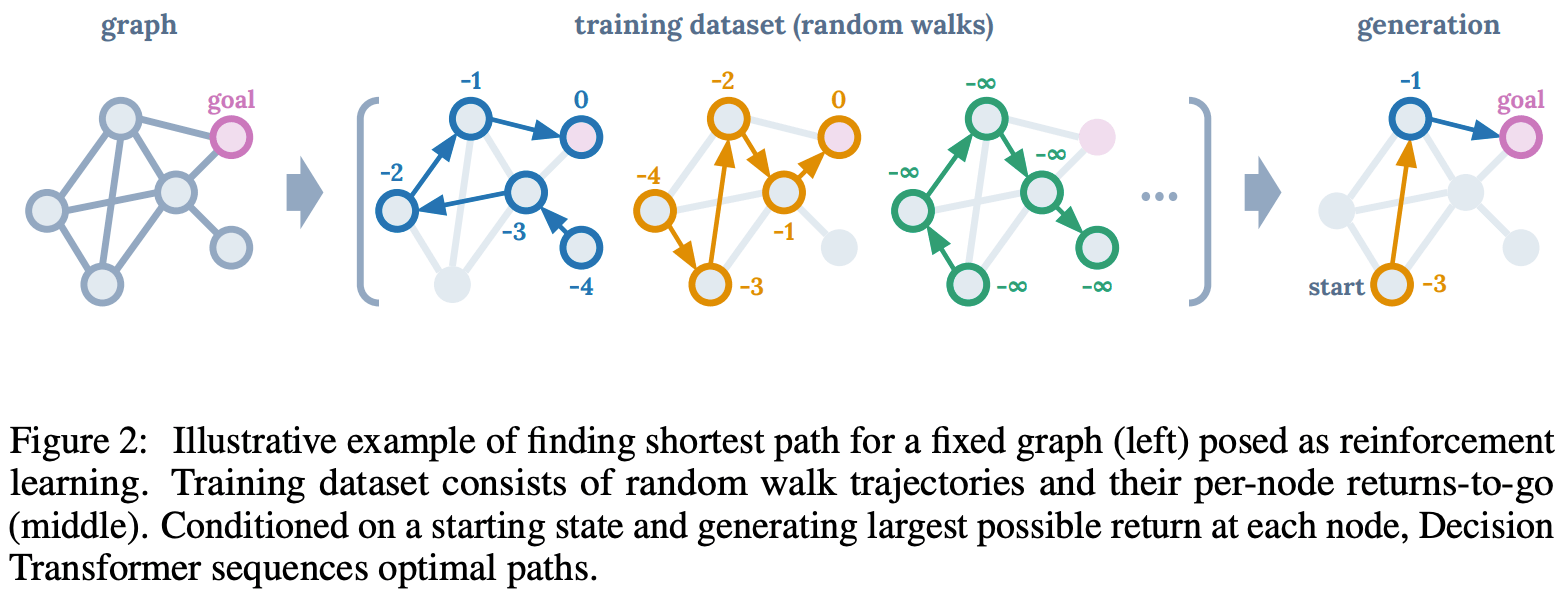## Proposed method: Decision Transformer

The architecture of the proposed method is quite simple. We consider a setting where the following trajectory series data are given. We consider the state and action at time t and the return-go-to as tokens and input the K tokens to the Causal Transformer of the GPT. The token is obtained by concatenating the convolutional layer (CNN) for the state S, and the return-to-go and action tokens through the linear layer.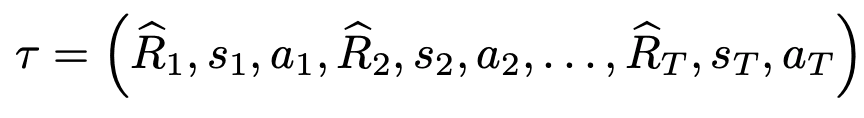Return-to-go:.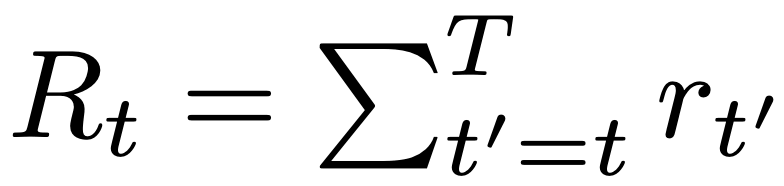During training, we first sample a mini-batch of series length = K from an offline dataset. Then, the tokens obtained from the information before time t are input to the Causal Transformer proposed in GPT, which is trained to predict the next action as an autoregressive model. In other words, it predicts the behavior at time t by inputting the state at time t, the return-go-to obtained after that time, and the past information, and predicts the next time using the predicted behavior.

Finally, it is trained with a cross-entropy loss function for discrete behaviors and with a mean squared error loss function for continuous behaviors.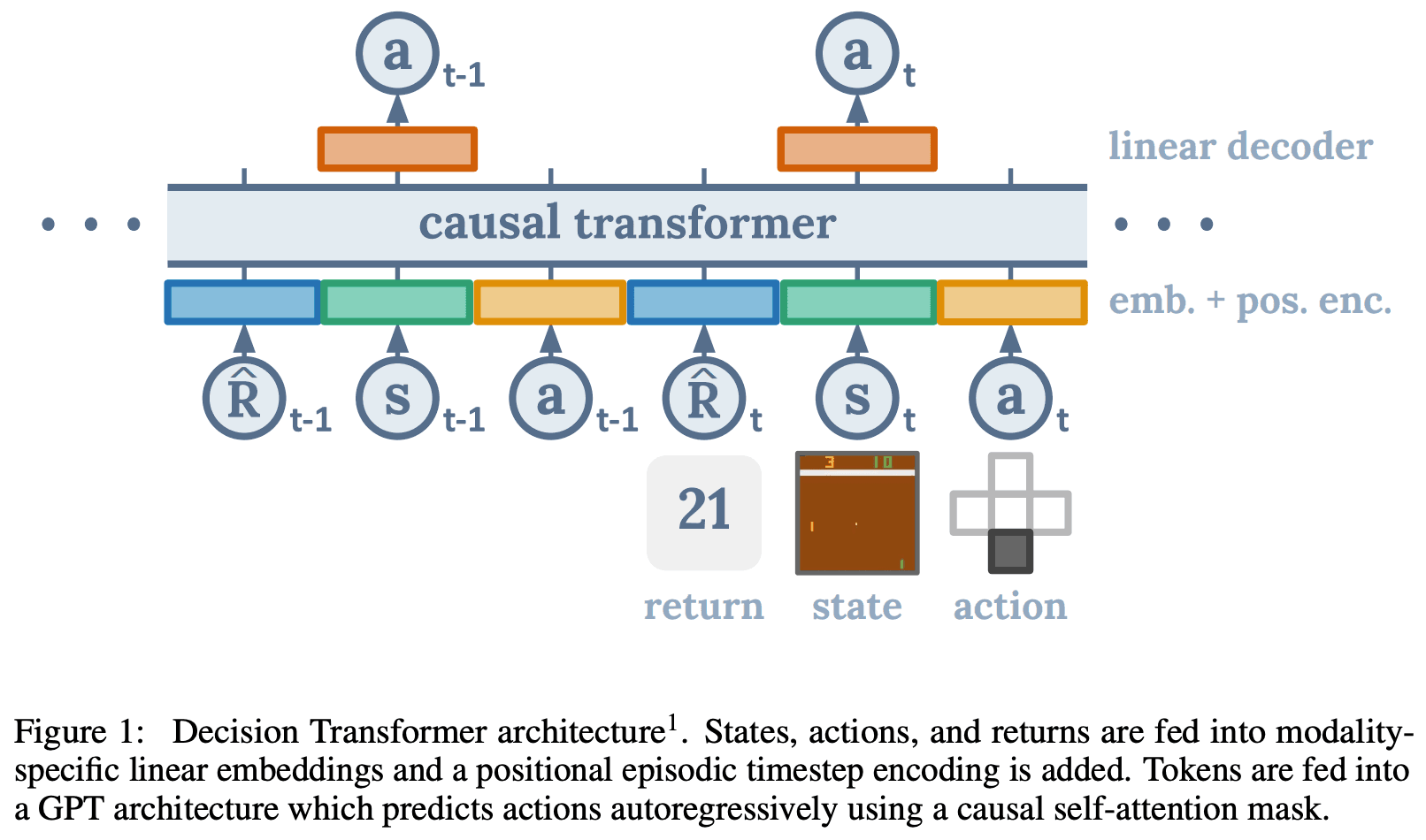However, during evaluation, the context length K and the target revenue are given as hyperparameters. The specific flow is shown in Algorithm 1.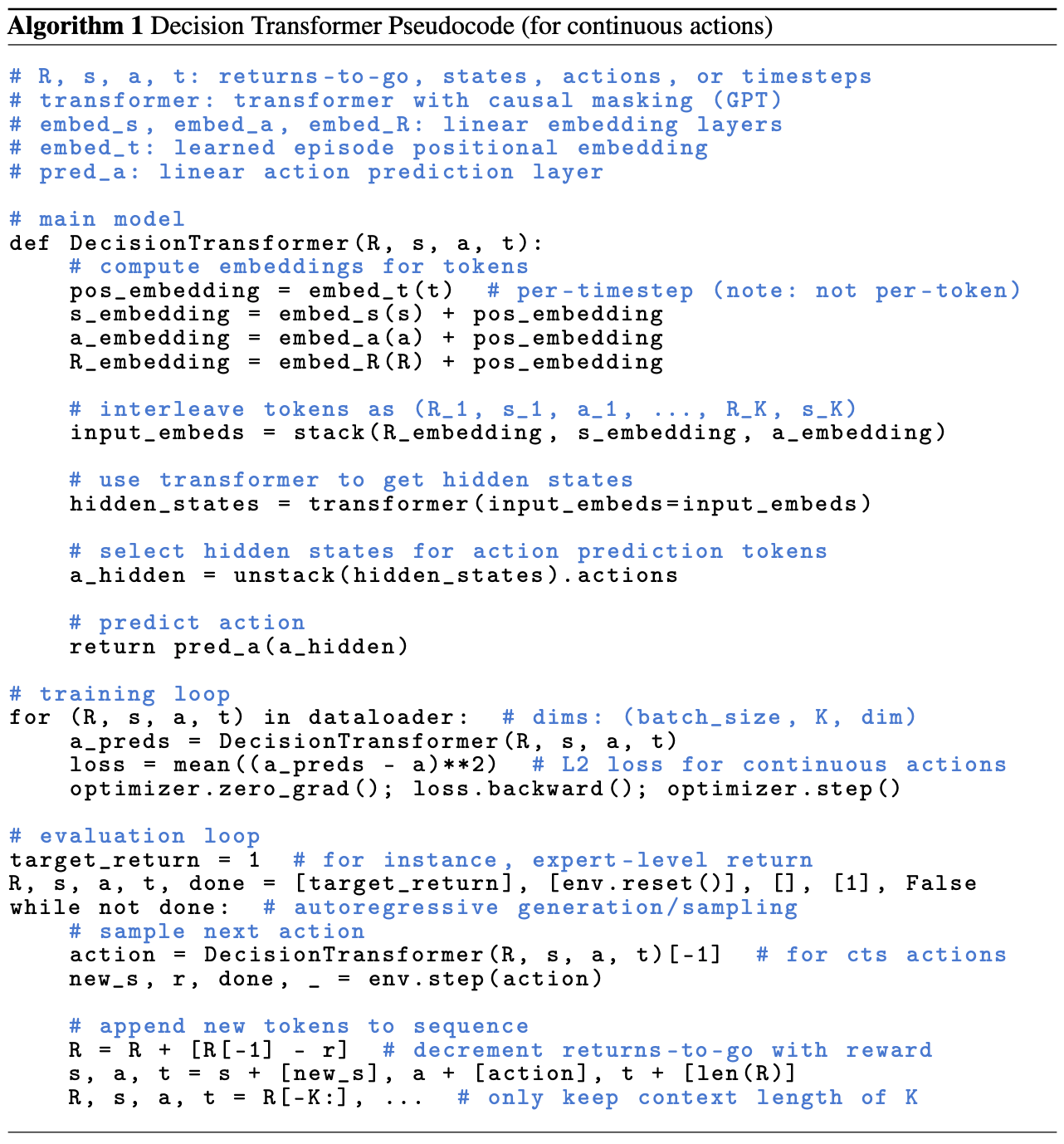## Experiments and Evaluation with Offline RL Benchmark

We chose a dedicated algorithm for Offline RL and Behavior Cloning (BCL) for the comparison method. Since the proposed Decision Transformer is a model-free method, we compared it with the model-free SOTA model Conservative Q-Learning (CQL), BEAR, and BRAC.

The experimental tasks were Atari, which has a discrete behavioral space that requires high-dimensional image observation and long-term credit allocation, and OpenAI Gym, which requires fine-grained continuous control.

Figure 3 shows that the accuracy of the Decision Transformer is comparable to that of the existing SOTA method in both experiments, indicating the proposed method's effectiveness.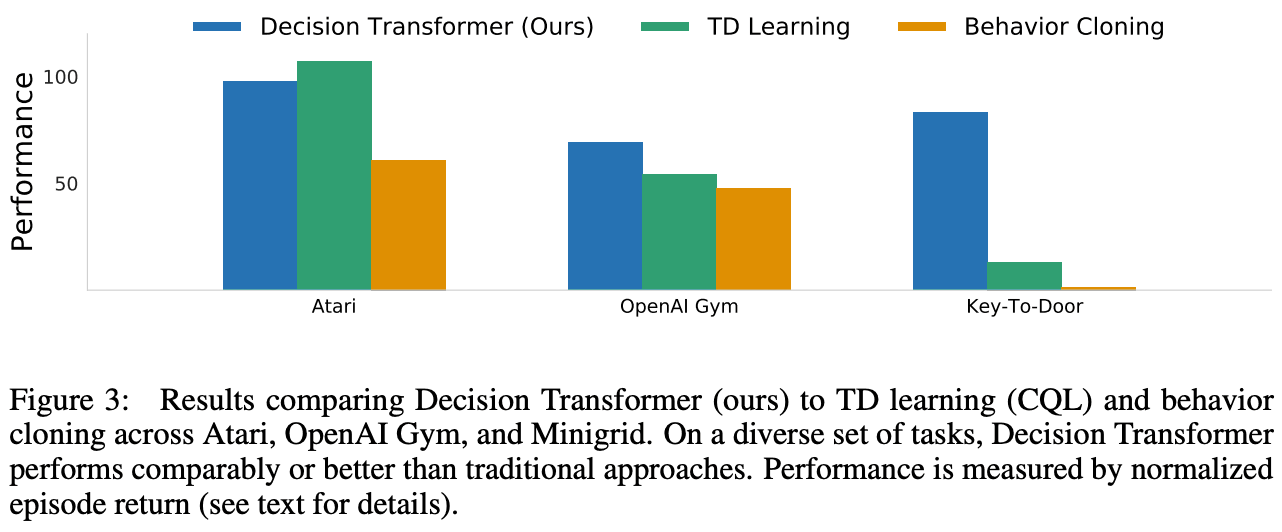### Atari (people)

We used 1% of the dataset consisting of trajectories of 5 million transactions obtained by the DQN strategy for training. We also introduced an evaluation where the pro-gamer's score was 100 and the random measure was 0.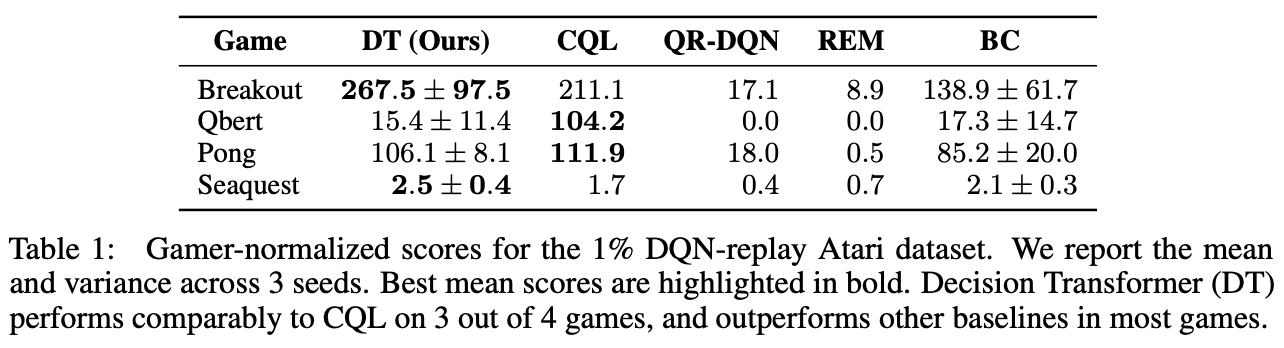We conducted evaluation experiments on four Atari tasks (Breakout, Qbert, Pong, and Seaquest) (Table 1), and found that the accuracy of our method was comparable to SOTA's CQL in three tasks and better than the other methods in all tasks.

### OpenAI Gym

In this session, we experimented with a 2D reacher environment in addition to the D4RL benchmark, which differs from the other tasks in that the rewards are discrete since the 2D reacher task is goal-directed. We used the three datasets described below.

1. medium: a dataset of 1 million timesteps collected by "medium-level" measures that reached 1/3 of the expert level using SAC in terms of score

2. Medium-Replay: all data sets stored in the buffer before reaching "medium level" in SAC

3. medium-expert: data set of 1 million timesteps collected by "medium-level" and "expert-level" measures, respectively

As shown in the Table 2 figure, we can compare the Model-free SOTA model CQL with all the recently proposed promising methods and see that the proposed method DT has better accuracy in most of the tasks.

OpenAI Gym was able to show the effectiveness of the proposed method more clearly than Atari.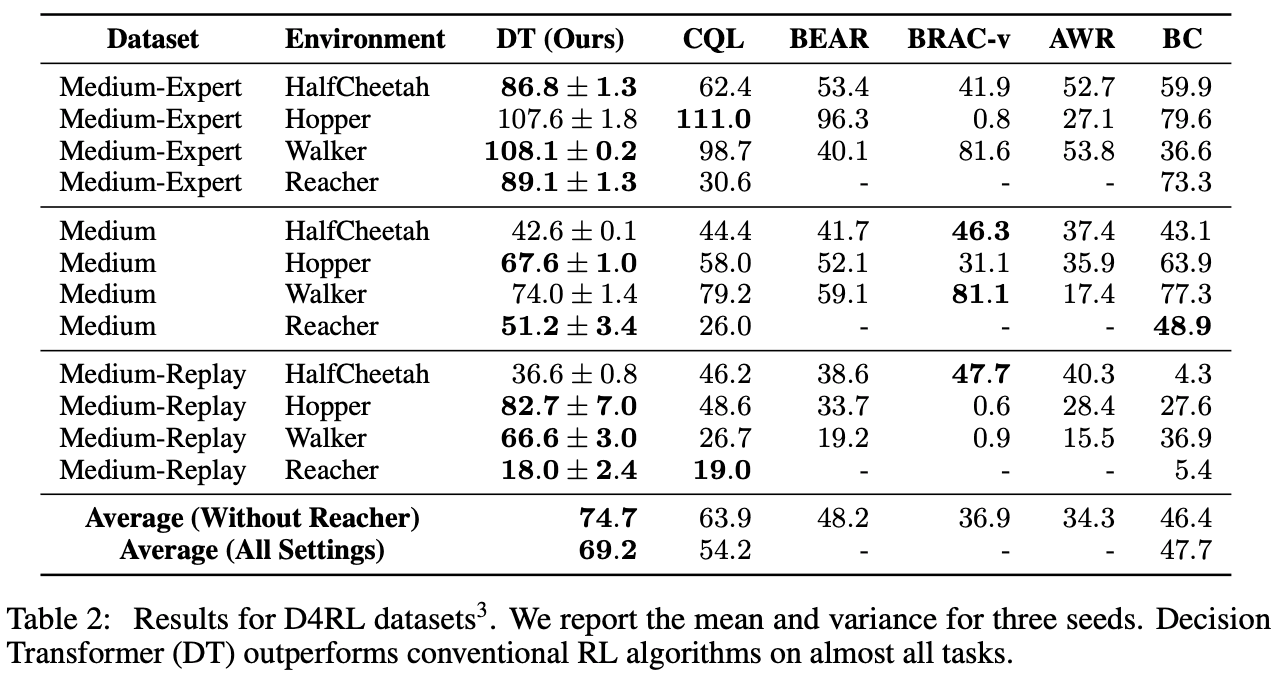## consideration

In this paper, we investigate the properties of the Decision Transformer by conducting some experiments. If you want to know the details, please refer to the paper.

In this section, we present the discussion from two perspectives: an analysis of the properties of the proposed Decision Transformer, such as how it differs from imitation learning, and how the objective revenue and context length K affect the results, and an analysis of the advantages that these properties provide in long series tasks.

### 1. how is Decision Transformer different from imitation learning in a setting where you get a specific revenue for some data sets?

The dataset was ordered by revenue per episode and Percentile Behavior Cloning (%BC), which was imitation trained using only the top X% of the data, was used to analyze how the proposed method differs from BC. The results show that Decision Transformer is as accurate as 10%BC. This indicates that the information to learn good strategies is concentrated in the top 10% of the dataset.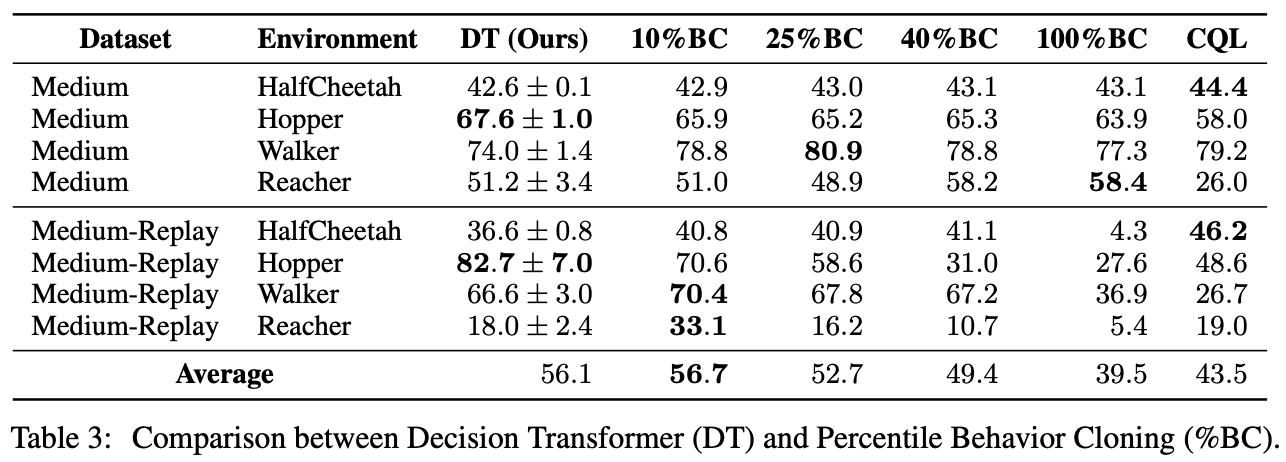On the other hand, in the experiment with Atari using only 1% of the data in the replay buffer, the accuracy of %BC was low and Decision Transformer was better. Therefore, it can be inferred that Decision Transformer efficiently selects the best data for imitation learning in the case of a small number of data.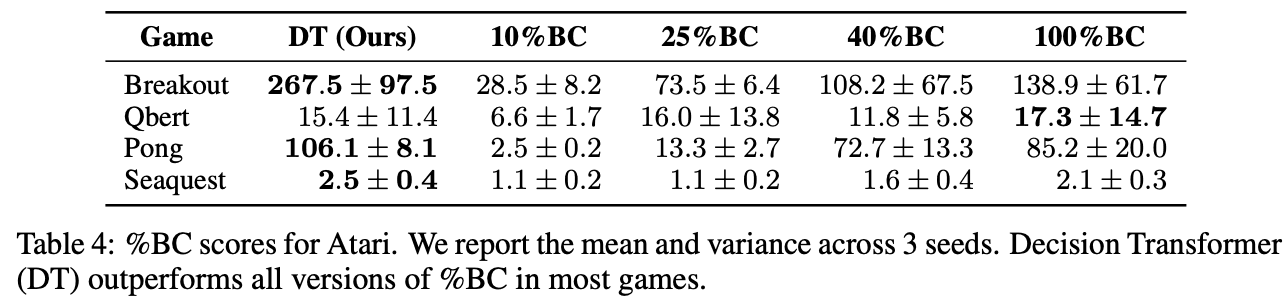### 2. the Decision Transformer predicts behavior based on the objective revenue and state, but how does that objective revenue affect the outcome?

We conducted an evaluation experiment by adjusting the objective reward setting to see if Decision Transformer understands the meaning of the revenue obtained after this.

As shown in Figure 4, for most tasks, the given objective revenue and the actual revenue obtained are correlated, indicating that the Decision Transformer can select appropriate actions depending on the objective revenue.

Furthermore, in Atari's Seaquest task, the fact that the revenue was higher than the highest revenue in the data set means that Decision Transformer has the ability to explore.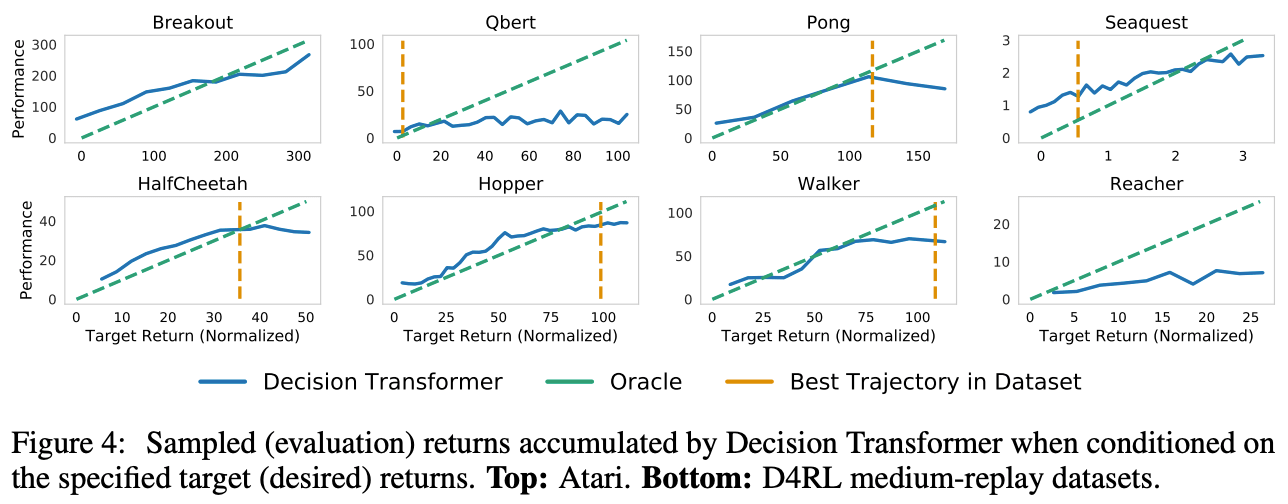### 3. how does the context length K of the Decision Transformer affect the results?

In general reinforcement learning using frame tracking, it has been thought that one previous state (K=1) is sufficient. However, in Decision Transformer, we have shown the importance of accessing the past information in our experiments, and it can be inferred that the past information is useful for Atari Game.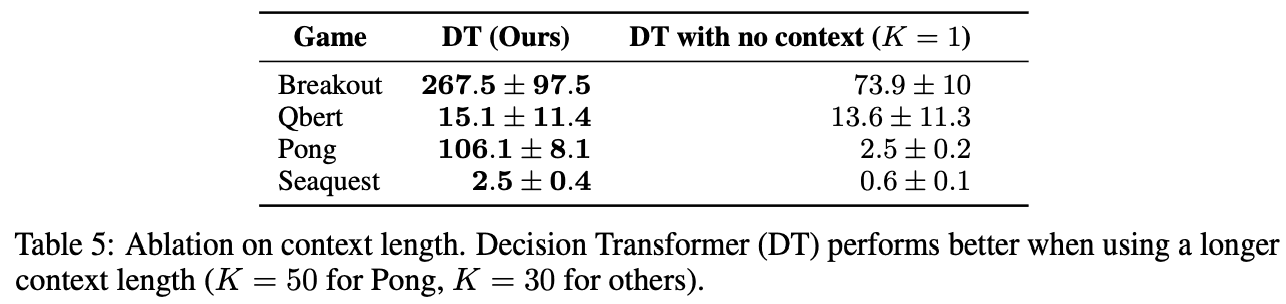### 4.Can Decision Transformer successfully deal with long-term credit distribution issues and sparse compensation settings?

How to deal with problem settings with long sequences has been a major challenge in reinforcement learning. Here, we consider a grid-based Key-to-Door environment where an agent takes an action in a room with a key, and then enters a room with a door via an empty room. If the agent does not acquire the key in the first room, it cannot open the door in the last hair and the task fails. We provide an empty room to evaluate the ability to distribute credits time over a long period of time.

In this experiment, we trained Decision Transformer on a dataset collected by randomly moving measures. We set the length of the context to be the episode length (K=episode length). We can see that only %BC and Decision Transformer, which mimic only the successful data, were able to learn the measures efficiently. In addition, TD Learning (CQL) produced low accuracy because it could not propagate Q-values over long time steps.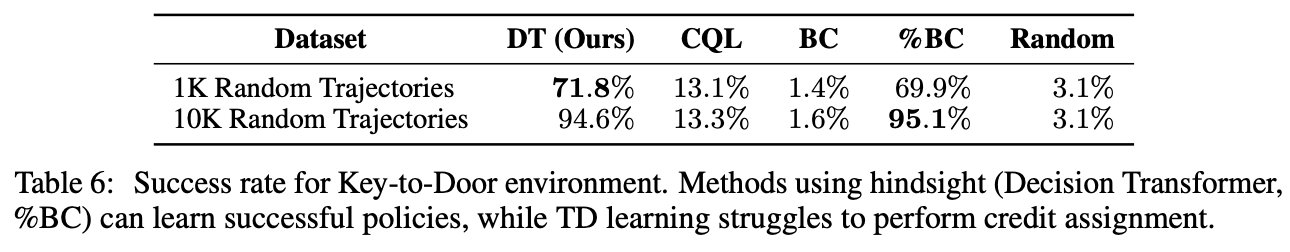Here, we modified the Decision Transformer to predict the reward along with the action to evaluate whether it can accurately capture the state. Figure 5 (left) shows that the Decision Transformer updates the predicted reward when the room changes. In addition, the attention weight is excited when the key is taken and the door is opened, indicating that the system is functioning correctly.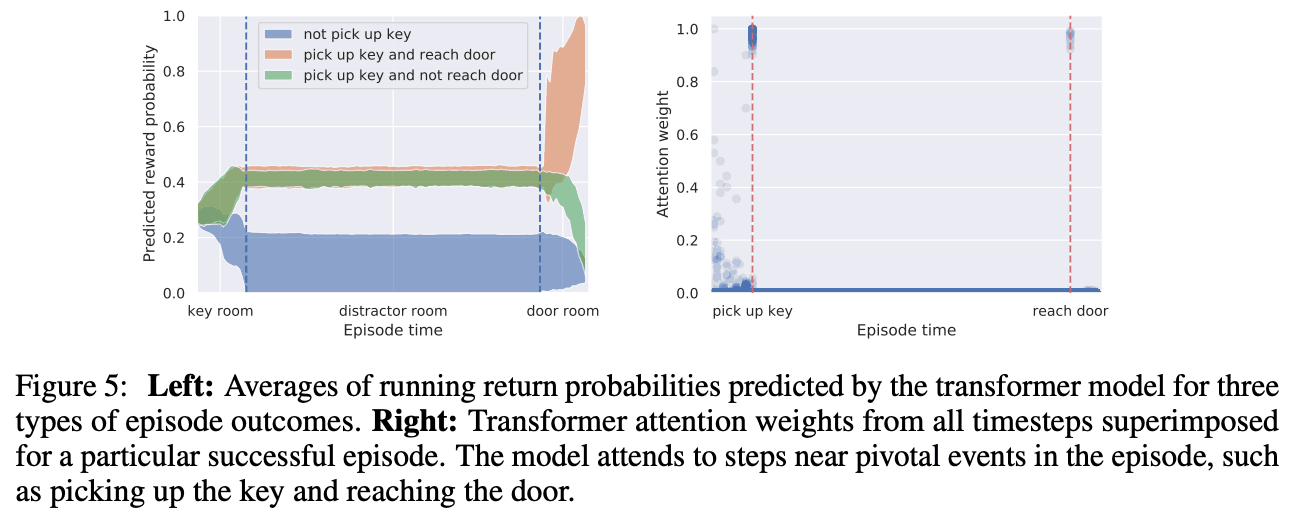## summary

In this article, we introduced Decision Transformer, which was motivated by the idea of adapting language sequence models to reinforcement learning. The Decision Transformer, which does not change the architecture of the language model in any way, performs as well as the Model-free SOTA method on the standard Offline RL benchmark.

Although it is a simple method with good results, we believe that the most important contribution of Decision Transformer is that it shows the possibility that reinforcement learning can also benefit from technological advances in natural language processing. In particular, the architecture of Decision Transformer is a natural fit for Offline RL, which aims to combine large-scale pre-learning and fine-tuning, and we hope that this research will lead to major reforms in reinforcement learning.

If you have any suggestions for improvement of the content of the article,